# 十个函数很简单，蹦蹦跳跳去加班

SUM

SUM函数的作用是求和。

=sum(A1:A10)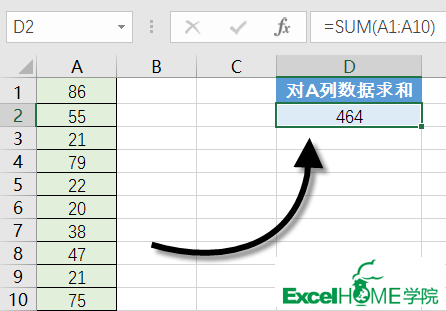=sum(A1:A10,C1:C10)

AVERAGE

Average 的作用是计算平均数。

=AVERAGE(A1:A10)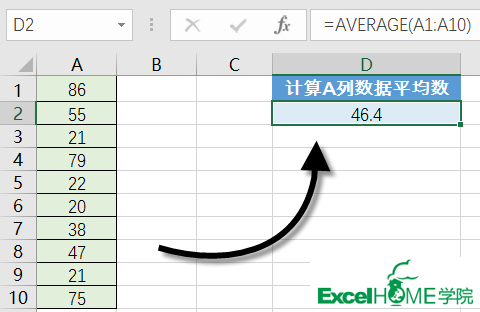=AVERAGE(A1:A10,D1:D10)

COUNT

COUNT函数计算含有数字的单元格的个数。

COUNT函数参数可以是单元格、单元格引用，或者数字。

COUNT函数会忽略非数字的值。

=COUNT(A1:A10)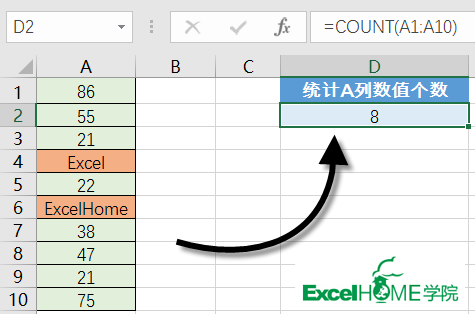IF

IF函数的作用是判断一个条件，然后根据判断的结果返回指定值。

IF函数的语法结构是：

=IF(逻辑判断,为TRUE时的结果,为FALSE时的结果)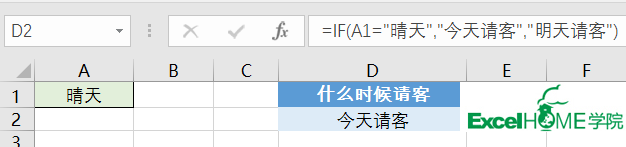NOW和TODAY

NOW函数返回日期和时间。TODAY函数则只返回日期。

=TODAY()-开始日期

NOW函数和TODAY函数都没有参数，只用一对括号即可：

=NOW()

=TODAY()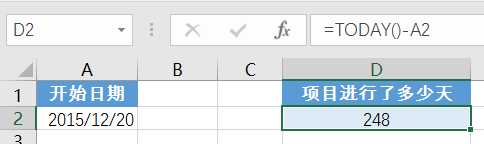ISNUMBER

ISNUMBER判断单元格中的值是否是数字，返回TRUE或FALSE。

=ISNUMBER(value)

MEDIAN函数
MEDIAN函数是返回一组数字的中值的。中值是啥？就是一组数的中间数啊。如果参数包含的数值个数是偶数，MEDIAN将返回位于中间的两个数的平均值。

=MEDIAN(C3:C10)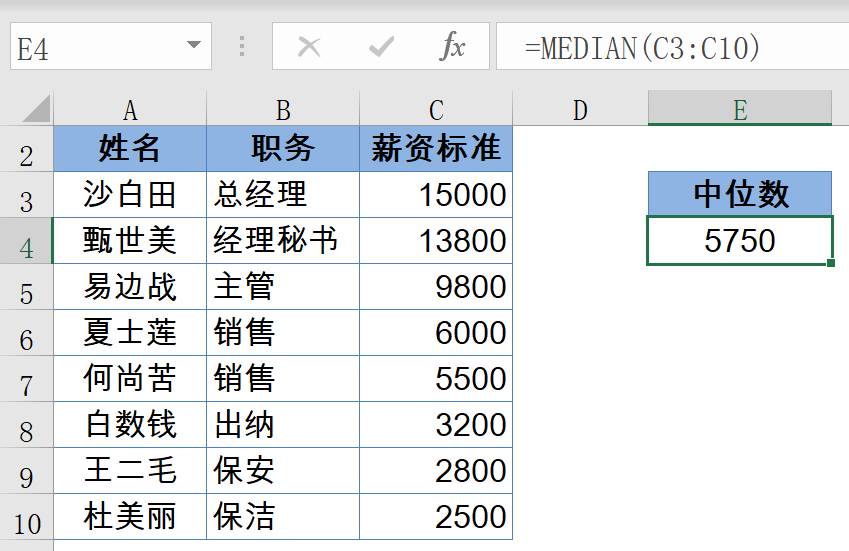EXACT函数
EXACT函数的作用是比较两个内容是否完全相同。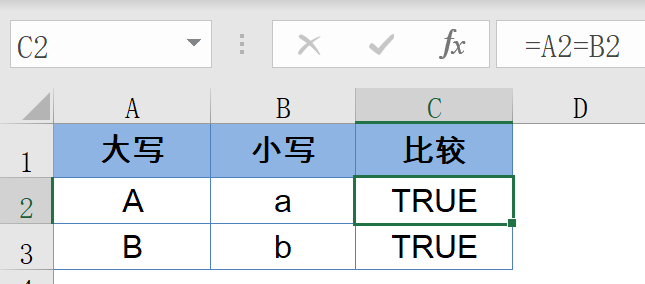=EXACT(A2,B2)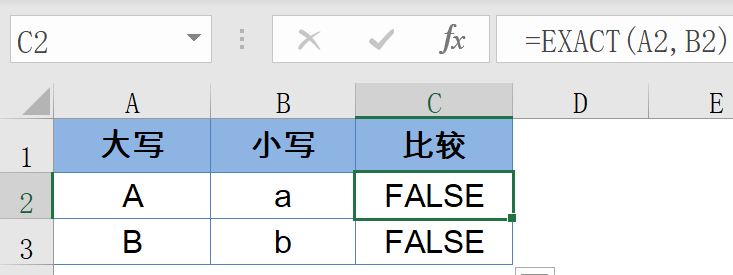SUBTOTAL函数

=SUBTOTAL(9,F2:F14)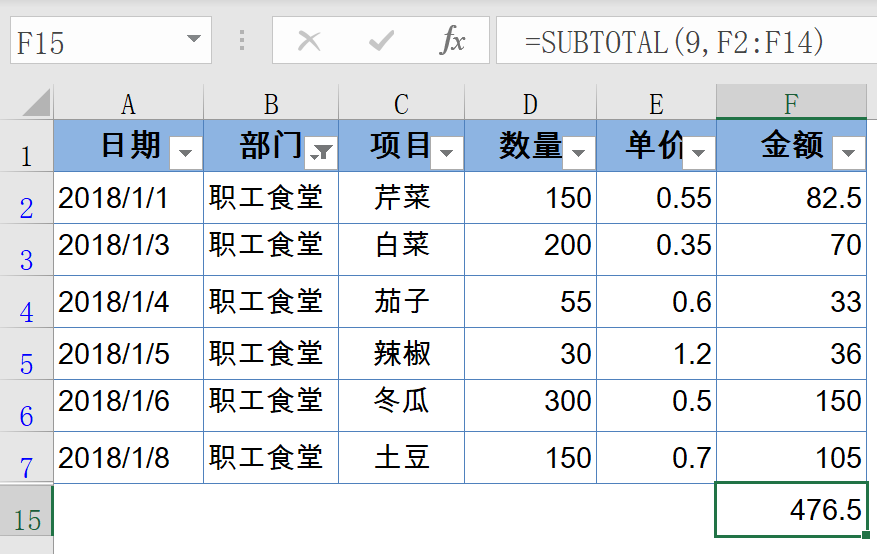SUBTOTAL第一参数用于指定汇总方式，可以是1~11的数值，通过指定不同的第一参数，可以实现平均值、求和、最大、最小、计数等多种计算方式。